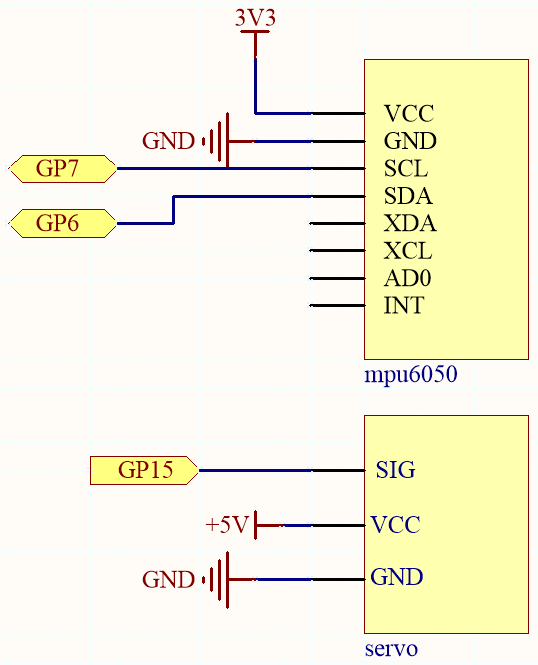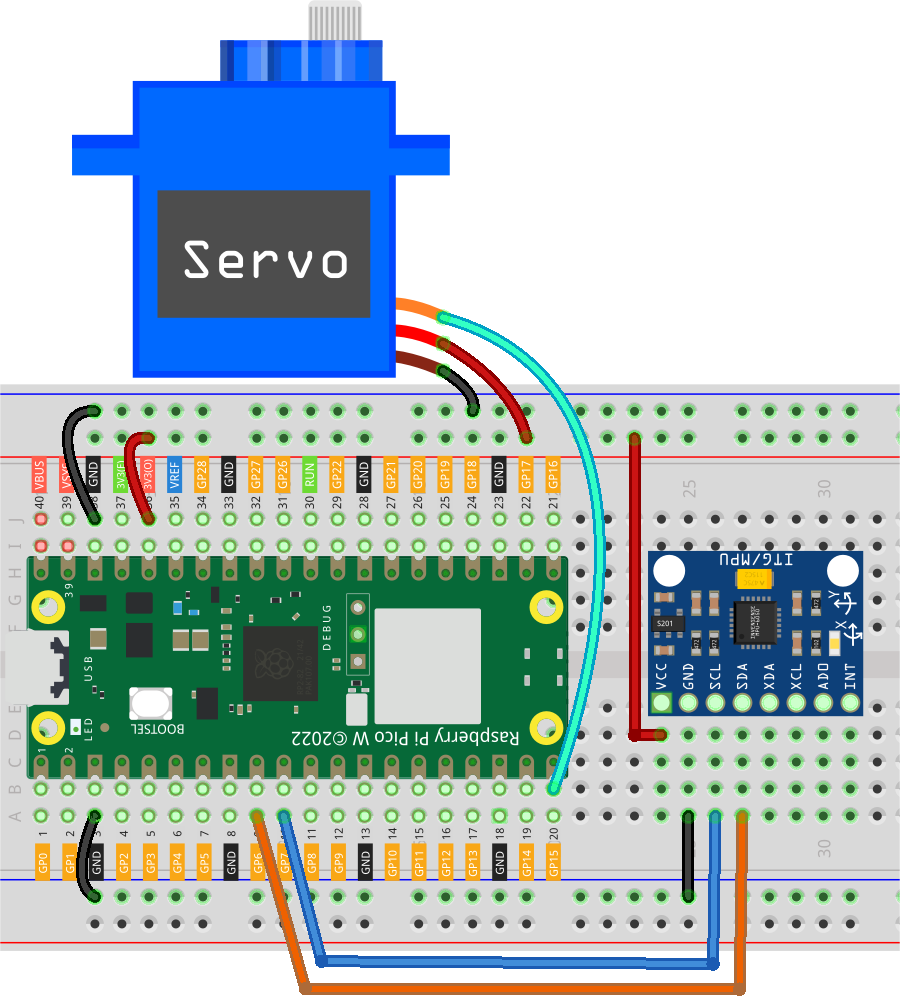# 7.11 Somatosensory Controller¶

If you watch a lot of robot movies, you’ve probably seen images like this. The protagonist turned his wrist and the giant robot followed; the protagonist shakes his fist, and the robot follows, which is very cool.

The use of this technology is already common in universities and research institutes, and the arrival of 5G will greatly expand its application areas. “Surgical robot da Vinci” remote surgery medical is a typical example.

A robotic system of this type is typically composed of two modules: a human motion capture module and a robotic arm actuation module (some application scenarios also include a data communication module).

The MPU6050 is used here to implement human motion capture (by mounting it on a glove) and the servo is used to represent robotic arm motion.

Required Components

In this project, we need the following components.

Name

ITEMS IN THIS KIT

Kepler Kit

450+

Kepler Kit

SN

COMPONENT

QUANTITY

1

Raspberry Pi Pico W

1

2

Micro USB Cable

1

3

1

4

Jumper Wires

Several

5

MPU6050 Module

1

6

Servo

1

SchematicThe MPU6050 calculates the attitude angle based on the acceleration values in each direction.

The program will control the servo to make the corresponding deflection angle as the attitude angle changes.

WiringCode

Note

• Open the `7.11_somatosensory_controller.py` file under the path of `kepler-kit-main/micropython` or copy this code into Thonny, then click “Run Current Script” or simply press F5 to run it.

• Don’t forget to click on the “MicroPython (Raspberry Pi Pico)” interpreter in the bottom right corner.

• For detailed tutorials, please refer to Open and Run Code Directly.

• Here you need to use the `imu.py` and `vector3d.py`, please check if it has been uploaded to Pico W, for a detailed tutorial refer to 1.4 Upload the Libraries to Pico.

```from imu import MPU6050
from machine import I2C, Pin
import time
import math

# mpu6050
i2c = I2C(1, sda=Pin(6), scl=Pin(7), freq=400000)
mpu = MPU6050(i2c)

# servo
servo = machine.PWM(machine.Pin(15))
servo.freq(50)

def interval_mapping(x, in_min, in_max, out_min, out_max):
return (x - in_min) * (out_max - out_min) / (in_max - in_min) + out_min

# get rotary angle
def dist(a,b):
return math.sqrt((a*a)+(b*b))

def get_y_rotation(x,y,z):

def get_x_rotation(x,y,z):

# servo work
def servo_write(pin,angle):
pulse_width=interval_mapping(angle, 0, 180, 0.5,2.5)
duty=int(interval_mapping(pulse_width, 0, 20, 0,65535))
pin.duty_u16(duty)

times=25
while True:
total=0
for i in range(times):
angle=get_y_rotation(mpu.accel.x, mpu.accel.y, mpu.accel.z) #get rotation value
total+=angle
average_angle=int(total/times) # make the value smooth
servo_write(servo,interval_mapping(average_angle,-90,90,0,180))
```

As soon as the program runs, the servo will turn left and right as you tilt the MPU6050 (or turn your wrist if it is mounted on a glove).